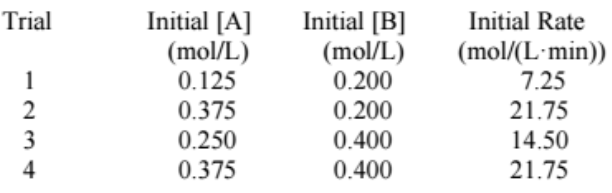# Problem: For the reaction A(g) + 2B(g) → 2C(g) + 2D(g), the following data was collected at constant temperature. Determine the correct rate law for this reaction. a) Rate = k[A][B] b) Rate = k[A]2[B] c) Rate = k[A][B]2 d) Rate = k[A] e) Rate = k[A]3

93% (58 ratings)
###### Problem Details

For the reaction A(g) + 2B(g) → 2C(g) + 2D(g), the following data was collected at constant temperature. Determine the correct rate law for this reaction.

a) Rate = k[A][B]

b) Rate = k[A]2[B]

c) Rate = k[A][B]2

d) Rate = k[A]

e) Rate = k[A]3What scientific concept do you need to know in order to solve this problem?

Our tutors have indicated that to solve this problem you will need to apply the Rate Law concept. You can view video lessons to learn Rate Law. Or if you need more Rate Law practice, you can also practice Rate Law practice problems.

What is the difficulty of this problem?

Our tutors rated the difficulty ofFor the reaction A(g) + 2B(g) → 2C(g) + 2D(g), the following...as high difficulty.

How long does this problem take to solve?

Our expert Chemistry tutor, Dasha took 7 minutes and 55 seconds to solve this problem. You can follow their steps in the video explanation above.

What professor is this problem relevant for?

Based on our data, we think this problem is relevant for Professor Roychowdhury's class at RUTGERS.